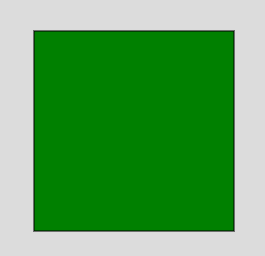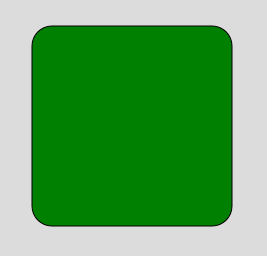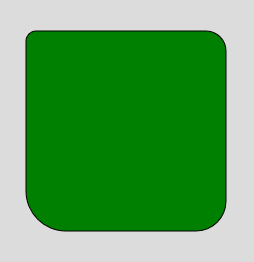# p5.js | square() Function

The square() function is an inbuilt function in p5.js which is used to draw the square on the screen. A square contains four equal sides and four angles each of 90 degrees. It is the special case of a rectangle where width and height are equal.

Syntax:

`square( x, y, s, tl, tr, br, bl )`

Parameters: This function accept many parameters as mentioned above and described below:

• x: It is used to set the x-coordinate of square.
• y: It is used to set the y-coordinate of square.
• s: It is used to set the size of side of square.
• tl: It is optional parameter and used to set the radius of top-left corner.
• tr: It is optional parameter and used to set the radius of top-right corner.
• br: It is optional parameter and used to set the radius of bottom-right corner.
• bl: It is optional parameter and used to set the radius of bottom-left corner.

Example 1:

 `function` `setup() {  ` `      `  `    ``// Create Canvas of given size  ` `    ``createCanvas(300, 300);  ` `  `  `}  ` `  `  `function` `draw() {  ` `      `  `    ``background(220); ` `      `  `    ``// Use color() function ` `    ``let c = color(``'green'``); ` `  `  `    ``// Use fill() function to fill color ` `    ``fill(c); ` `    `  `    ``// Draw a square ` `    ``square(50, 50, 200); ` `    `  `}  `

Output:Example 2:

 `function` `setup() {  ` `      `  `    ``// Create Canvas of given size  ` `    ``createCanvas(300, 300);  ` `  `  `}  ` `  `  `function` `draw() {  ` `      `  `    ``background(220); ` `      `  `    ``// Use color() function ` `    ``let c = color(``'green'``); ` `  `  `    ``// Use fill() function to fill color ` `    ``fill(c); ` `    `  `    ``// Draw a square ` `    ``square(50, 50, 200, 20); ` `    `  `}  `

Output:Example 3:

 `function` `setup() {  ` `      `  `    ``// Create Canvas of given size  ` `    ``createCanvas(300, 300);  ` `  `  `}  ` `  `  `function` `draw() {  ` `      `  `    ``background(220); ` `      `  `    ``// Use color() function ` `    ``let c = color(``'green'``); ` `  `  `    ``// Use fill() function to fill color ` `    ``fill(c); ` `    `  `    ``// Draw a square ` `    ``square(50, 50, 200, 10, 20, 30, 40); ` `    `  `}  `

Output:Online editor: https://editor.p5js.org/
Environment Setup: https://www.geeksforgeeks.org/p5-js-soundfile-object-installation-and-methods/

Reference: https://p5js.org/reference/#/p5/squareMy Personal Notes arrow_drop_upCheck out this Author's contributed articles.

If you like GeeksforGeeks and would like to contribute, you can also write an article using contribute.geeksforgeeks.org or mail your article to contribute@geeksforgeeks.org. See your article appearing on the GeeksforGeeks main page and help other Geeks.

Please Improve this article if you find anything incorrect by clicking on the "Improve Article" button below.

Article Tags :

Be the First to upvote.

Please write to us at contribute@geeksforgeeks.org to report any issue with the above content.